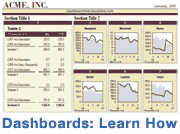How do you tell Excel to round to a specific number of significant figures without having to use exponential notation? This Excel formula will do the trick:

`=ROUND(value,sigfigs-(1+INT(LOG10(ABS(value)))))`

value :: the number you wish to round.
sigfigs :: the number of significant figures you want to round to.

There. Quick and easy. Continue reading below for more explanation about how this formula works, and visit my Excel Tips and Excel Templates pages for a lot of other great resources.

### How the formula works:

The trick to this formula comes from understanding scientific notation. Reporting the number 12783 with three significant digits would give 1.28E4 or 1.28*10^4 or base*10^exponent.

Let's work backwards from what we want. We want to use the ROUND function for starters. But, we need to know the "location" of the digit to round to. Remember that the way the ROUND function works in Excel, rounding 12783 to the 100s place means you use a "location" of -2 or 12800=ROUND(12783,-2). If we want 3 significant digits, we just need to create a formula that gives -2 based upon the position of the first significant digit, or 1+exponent.

The formula for the exponent of 12783 is:

`4 =INT(LOG10(ABS(12783)))`

There we have it: 3 - (1 + 4) = -2

You can also use the ROUNDDOWN or ROUNDUP function in place of the ROUND function.

### Custom Function for Rounding to Significant Figures

Syntax: ROUNDSIG(value,sigfigs)

Example: ROUNDSIG(-0.04589,2) equals -0.046

```Function ROUNDSIG(num As Variant, sigs As Variant)
Dim exponent As Double
If IsNumeric(num) And IsNumeric(sigs) Then
If sigs < 1 Then
' Return the  " #NUM "  error
ROUNDSIG = CVErr(xlErrNum)
Else
If num <> 0 Then
exponent = Int(Log(Abs(num)) / Log(10#))
Else
exponent = 0
End If
ROUNDSIG = WorksheetFunction.Round(num, _
Sigs - (1 + exponent))
End If
Else
' Return the  " #N/A "  error
ROUNDSIG = CVErr(xlErrNA)
End If
End Function
```

See Also: "How to Create Custom Excel Functions"

Note: It has been correctly pointed out (here) that trailing zeros on decimals numbers don't necessarily display correctly when using the above formulas. Although the value will be correct, Excel automatically formats a number with 5 sig figs such as 23.300 to display as 23.3 (unless the display format has been set to "0.000").

### Working Around the Display Format Problem

To ensure that significant trailing zeros are displayed correctly when rounding a number to a certain number of significant digits, you need to work with text formats. The following formula is very confusing, but it gets the job done.

Update 4/19/2016: Fix for numbers such as 99.95 through 99.9999999 rounded to 3 SDs:

Note: If you are okay with leaving the number displayed in a scientific notation (e.g. 0.100E+003 for the number 100), then the formula can be as simple as this:

Another Note: You can convert a text number to a value by multiplying by 1 or adding 0 or using double negation, for example:

I got the idea to use the text format in this way from John McGimpsey's site. However, the above megaformula lets you choose any number of significant digits. Below is a VBA function using this method to round significant digits. The function returns the value as a string, so when using the value in other formulas, you can use VALUE(cell) to convert the string to a numeric value.

```Function ROUNDSF(num As Variant, sigs As Variant) As String
Dim exponent As Integer
Dim decplace As Integer
Dim fmt_left As String
Dim fmt_right As String
Dim numround As Double
If IsNumeric(num) And IsNumeric(sigs) Then
If sigs < 1 Then
' Return the   "  #NUM  "   error
ROUNDSF = CVErr(xlErrNum)
Else
numround = WorksheetFunction.text(num, "." & _
String(sigs, "0") & "E+000")
If num = 0 Then
exponent = 0
Else
'Round is needed to fix a ?truncation?
'problem when num = 10, 100, 1000, etc.
exponent = Round(Int(Log(Abs(numround)) / Log(10)), 1)
End If
decplace = (sigs - (1 + exponent))
If decplace > 0 Then
fmt_right = String(decplace, "0")
fmt_left = "0."
Else
fmt_right = ""
fmt_left = "0"
End If
ROUNDSF = WorksheetFunction.text(numround, _
fmt_left & fmt_right)
End If
Else
' Return the   "  #N/A  "   error
ROUNDSF = CVErr(xlErrNA)
End If
End Function
```

### References

• Eric W. Weisstein. "Significant Digits." From MathWorld--A Wolfram Web Resource. http://mathworld.wolfram.com/SignificantDigits.html
• iSigSigma.com, "Rounding Rules and Round-Off Rules for Statistical Data Analyses," http://www.isixsigma.com/library/content/t000813.asp
• "Calculations with Significant Figures." http://www.mcgimpsey.com